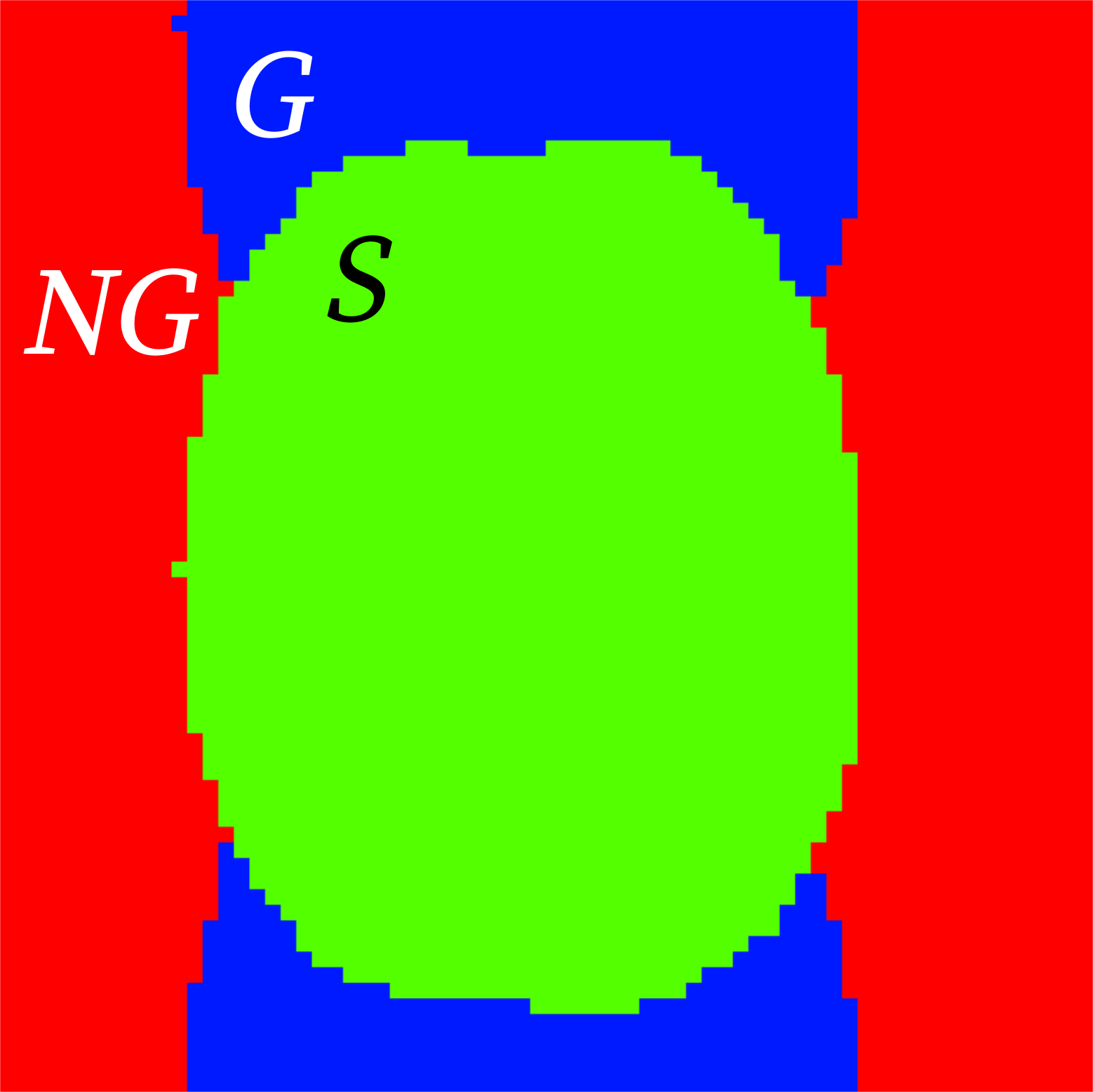# IQMs for functional images¶

## Measures for the spatial information¶

Definitions are given in the summary of structural IQMs.

• Full-width half maximum smoothness (fwhm_*, see [Friedman2008]).

### Measures for the temporal information¶

DVARS

D referring to temporal derivative of timecourses, VARS referring to RMS variance over voxels ([Power2012] dvars_nstd) indexes the rate of change of BOLD signal across the entire brain at each frame of data. DVARS is calculated with nipype after motion correction:

$\text{DVARS}_t = \sqrt{\frac{1}{N}\sum_i \left[x_{i,t} - x_{i,t-1}\right]^2}$

Note

Intensities are scaled to 1000 leading to the units being expressed in x10 $$\%\Delta\text{BOLD}$$ change.

Note

MRIQC calculates two additional standardized values of the DVARS. The dvars_std metric is normalized with the standard deviation of the temporal difference time series. The dvars_vstd is a voxel-wise standardization of DVARS, where the temporal difference time series is normalized across time by that voxel standard deviation across time, before computing the RMS of the temporal difference [Nichols2013].

Global Correlation (gcor)

calculates an optimized summary of time-series correlation as in [Saad2013] using AFNI’s @compute_gcor:

$\text{GCOR} = \frac{1}{N}\mathbf{g}_u^T\mathbf{g}_u$

where $$\mathbf{g}_u$$ is the average of all unit-variance time series in a $$T$$ (# timepoints) $$\times$$ $$N$$ (# voxels) matrix.

Temporal SNR (tSNR, tsnr)

is a simplified interpretation of the tSNR definition [Kruger2001]. We report the median value of the tSNR map calculated like:

$\text{tSNR} = \frac{\langle S \rangle_t}{\sigma_t},$

where $$\langle S \rangle_t$$ is the average BOLD signal (across time), and $$\sigma_t$$ is the corresponding temporal standard-deviation map. Higher values are better.

### Measures for artifacts and other¶

Framewise Displacement

expresses instantaneous head-motion [Jenkinson2002]. MRIQC reports the average FD, labeled as fd_mean. Rotational displacements are calculated as the displacement on the surface of a sphere of radius 50 mm [Power2012]:

$\text{FD}_t = |\Delta d_{x,t}| + |\Delta d_{y,t}| + |\Delta d_{z,t}| + |\Delta \alpha_t| + |\Delta \beta_t| + |\Delta \gamma_t|$

Along with the base framewise displacement, MRIQC reports the number of timepoints above FD threshold (fd_num), and the percent of FDs above the FD threshold w.r.t. the full timeseries (fd_perc). In both cases, the threshold is set at 0.20mm.

Ghost to Signal Ratio (gsr())

labeled in the reports as gsr_x and gsr_y (calculated along the two possible phase-encoding axes x, y):

$\text{GSR} = \frac{\mu_G - \mu_{NG}}{\mu_S}$AFNI’s outlier ratio (aor)

Mean fraction of outliers per fMRI volume as given by AFNI’s 3dToutcount.

AFNI’s quality index (aqi)

Mean quality index as computed by AFNI’s 3dTqual; for each volume, it is one minus the Spearman’s (rank) correlation of that volume with the median volume. Lower values are better.

Number of dummy scans** (dummy)

A number of volumes in the begining of the fMRI timeseries identified as non-steady state.

References

Atkinson1997

Atkinson et al., Automatic correction of motion artifacts in magnetic resonance images using an entropy focus criterion, IEEE Trans Med Imag 16(6):903-910, 1997. doi:10.1109/42.650886.

Friedman2008

Friedman, L et al., Test–retest and between‐site reliability in a multicenter fMRI study. Hum Brain Mapp, 29(8):958–972, 2008. doi:10.1002/hbm.20440.

Giannelli2010

Giannelli et al., Characterization of Nyquist ghost in EPI-fMRI acquisition sequences implemented on two clinical 1.5 T MR scanner systems: effect of readout bandwidth and echo spacing. J App Clin Med Phy, 11(4). 2010. doi:10.1120/jacmp.v11i4.3237.

Jenkinson2002

Jenkinson et al., Improved Optimisation for the Robust and Accurate Linear Registration and Motion Correction of Brain Images. NeuroImage, 17(2), 825-841, 2002. doi:10.1006/nimg.2002.1132.

Kruger2001

Krüger et al., Physiological noise in oxygenation-sensitive magnetic resonance imaging, Magn. Reson. Med. 46(4):631-637, 2001. doi:10.1002/mrm.1240.

Nichols2013

Nichols, Notes on Creating a Standardized Version of DVARS, 2013.

Power2012(1,2)

Power et al., Spurious but systematic correlations in functional connectivity MRI networks arise from subject motion, NeuroImage 59(3):2142-2154, 2012, doi:10.1016/j.neuroimage.2011.10.018.

Saad et al. Correcting Brain-Wide Correlation Differences in Resting-State FMRI, Brain Conn 3(4):339-352, 2013, doi:10.1089/brain.2013.0156.

mriqc.qc.functional.gsr(epi_data, mask, direction='y', ref_file=None, out_file=None)[source]

Compute the GSR [Giannelli2010].

The procedure is as follows:

1. Create a Nyquist ghost mask by circle-shifting the original mask by $$N/2$$.

2. Rotate by $$N/2$$

3. Remove the intersection with the original mask

4. Generate a non-ghost background

5. Calculate the GSR

Warning

This should be used with EPI images for which the phase encoding direction is known.

Parameters
• epi_file (str) – path to epi file